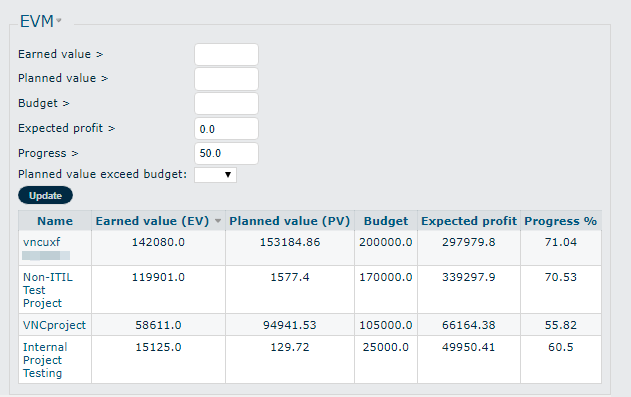# VNCproject Earned Value Management (EVM)¶

## Quick overview of Earned Value Management¶

Earned Value Management (EVM) is a project-controlling technique that objectively tracks the physical accomplishment of work and predicts the future performance of the project.

• EVM is used to measure the progress and to assess the status of a project to enable timely, effective management decisions.
• EVM integrates technical scope with schedule and cost objectives during the execution of the project.
• EVM provides not only the past and the current performance of a project but also forecasts its likely future performance.
• EVM answers a lot of questions to the stakeholders in a project related to its performance.
• Good planning coupled with effective use of EVM will reduce a lot of issues arising out of schedule and cost overruns.

## The three basic factors: Planned Values, Actual Cost, Earned Value¶

### Planned Value (PV)¶

Planned value (PV) is also referred to as the Budgeted Cost of Work Scheduled (BCWS). PV or BCWS is the total cost of the work scheduled/planned as of a reporting period. This period could represent days, weeks, months, etc. It is calculated as:

PV or BCWS = User's Hourly Rate × Total Hours Estimated + Other Planned Costs

### Actual Cost (AC)¶

Actual cost (AC) is also referred to as the Actual Cost of Work Performed (ACWP). AC or ACWP is the total cost taken to complete the work as of a reporting period. This period could represent days, weeks, months, etc. It is calculated as:

AC or ACWP =User's Hourly Rate × Total Hours Spent + Other Actual Costs

### Earned Value (EV)¶

Earned value (EV) is also referred to as the Budgeted Cost of Work Performed (BCWP). EV or BCWP is the total cost of the work completed/performed as of a reporting period. This period could represent days, weeks, months, etc. It is calculated as:

EV or BCWP = Planned Value × % Complete Actual (% Progress)

## Factors to measure the actual performance of a project¶

### Cost Variance (CV)¶

A metric for the cost performance derived from earned value data. It may be expressed as a value for a specific period of time or cumulative to date. CV gives the variance in terms of cost and indicates how much over - or under-budget the project is. It is the algebraic difference between earned value and actual cost and can be calculated using the following formula:

Cost Variance (CV) = Earned Value (EV) − Actual Cost (AC)

• A positive CV indicates a favorable condition: the project is under-budget.
• A negative CV indicates an unfavorable condition: the project is over-budget.

### Cost Performance Indicator (CPI)¶

The Cost Performance Indicator (CPI) is an index showing the cost efficiency of the project. The CPI compares the earned value to the actual cost to perform that work. It can be calculated using the following formula:

CPI = Earned Value (EV) ⁄ Actual Cost (AC)

• A CPI of 1.0 means that we are spending exactly what we planned to spend to accomplish the work performed. (on the budget)
• A CPI above 1.0 means we are under running costs. Efficiency is realized (under budget)
• A CPI below 1.0 means that we are overrunning costs. Efficiency is low (over budget)

### Schedule Variance (SV)¶

A metric for the schedule performance derived from earned value data. It may be expressed for a specific period of time or cumulative to date. SV gives the variance in terms of cost which indicates how much cost of the work is yet to be completed as per schedule or how much ahead or behind the schedule a project is running. It is the algebraic difference between earned value and the planned value and can be calculated using the following formula:

Schedule Variance (SV) = Earned Value (EV) − Planned Value (PV)

• A positive SV indicates a favorable condition: we are ahead of schedule.
• A negative SV indicates an unfavorable condition: we are behind schedule.

### Schedule Performance Indicator (SPI)¶

The Schedule Performance Indicator (SPI) is an index showing the time efficiency of the project. The SPI compares the earned value to the work scheduled. It can be calculated using the following formula:

SPI = Earned Value (EV) ⁄ Planned Value (PV)

• An SPI of 1.0 means the work is being performed right to the schedule. (on schedule).
• An SPI above 1.0 means that the work is ahead of schedule. Efficiency is realized.
• An SPI below 1.0 means that the work is behind schedule. Efficiency is low.

## Factors to forecast the future performance of a project¶

### Budget at Completion (BAC)¶

Budget at Completion (BAC) or the project cost baseline is the total of all budgets established for the work to be performed. This is the “contract sale price” for the project’s deliverables paid by the customer. In commercial contracts, this includes the performing organization’s profit margin and any other fees and charges that the organization will recover from the customer. In a project, the BAC is the contract value excluding these profits. It can be calculated using the following formula:

Budget at Completion (BAC) = Contract Value - Desired Profit

• The BAC is generally plotted over time, for example, periods of reporting (monthly, weekly, etc.). So that means the budgets for all authorized work must be captured within the BAC.
• The BAC also can be referred to as the total cumulative planned value (PV) of all periods. When all of the work on the project is 100% complete: BAC = Planned Value = Earned Value All three of these values are derived from the authorized budget for the work
• The BAC is used to compute the Estimate at Completion (EAC) and explained in the next section.

### Estimate at Completion (EAC)¶

The Estimate at Completion (EAC) is an independent forecast of the total cost at the completion of the project. So it is used to predict future cost performance for all authorized work.

There are many different options for calculating EAC and depending on the situation presented we can choose the appropriate one to use:

1. The calculation in the first EAC formula uses the Budget at Completion (BAC) and the Cost Performance (CPI) Index to calculate the EAC: (TYPICAL)

EAC = BAC / CPI

• This method assumes what the project has experienced to date can be expected to continue in the future. So the overall budget is scaled in proportion to the cost performance to date. (BAC divided by CPI).
• This is a realistic method, which is appreciated by many experts in project management.

• The second EAC formula is used to scale future cost performance based on actual performance to date:

EAC = AC + [(BAC - EV) / CPI]

• This method is used when the assumption is that the original estimates were solid but the current variances will continue to be present in the future. The total estimate is the actual cost to date plus the remaining budget scaled in proportion to the cost performance to date. (Actual Cost plus the remaining budget divided by CPI)
• If there has been no change in the project, this is mathematical, identical to the formula above. However, if the project has been rebaselined, this formula is more useful.
• This tends to be the lowest likely cost outcome if the project is over budget (Low-End Cumulative CPI EAC).

• In the third EC formula, there is an assumption that the future performance will be accomplished with the original budget:

EAC = AC + (BAC - EV)

• The total estimate is the actual cost to date plus the remaining budget (BAC - EV).
• This method is used early in every project to avoid major fluctuations. In this case, the variances at the current stage are typical (through to around the 15% to 20% stage) and are not expected to occur in the future in any circumstances.

• In this forecast, future performance will be performed at an efficiency rate that combines both the cost and schedule performance indices:

EAC = AC + [(BAC - EV) / (CPI × SPI)]

• The total estimate is the actual cost to date plus the remaining budget scaled in proportion to the cost performance to date, adjusted by SPI. This method is most useful when the schedule performance has an impact on cost performance.
• This tends to be the highest likely cost outcome if the project is over budget and over time (High-End Cumulative CPI × SPI EAC).

• There is a method, which is used when the past estimating assumptions are not valid and fresh estimates are applied to the project:

EAC = AC + Bottom-Up Estimate to Completion (ETC)

In VNCproject, the EAC is calculated with the first formula.

### Variance at Completion (VAC)¶

Variance at completion (VAC) is the variance in the total budget at the end of the project. This is the difference between what the project was originally expected baseline to cost versus what it is now expected to cost.

The VAC is calculated using the following formula:

VAC = BAC – EAC

• If the result is POSITIVE, the project is projecting an “Underrun”
• If the result is NEGATIVE, the project is projecting an “Overrun”

In VNCproject, the VAC is used as “Expected Profit” and is calculated as the formula below:

VAC = Expected Profit = Contract Value – EAC

## How to use the EVM module¶

To use the earned value method we need to identify the following inputs:

### Define an hourly rate for the employee¶

1. In the navigation menu, click the kebab menu icon.
3. Click Users. Select a required user.
4. Under the Information section, you can define two kinds of hourly rates for an employee:

• Hourly rate is used for the internal projects;
• Customer hourly rate is used for all projects with project type “Customer Project”.
5. Click Save.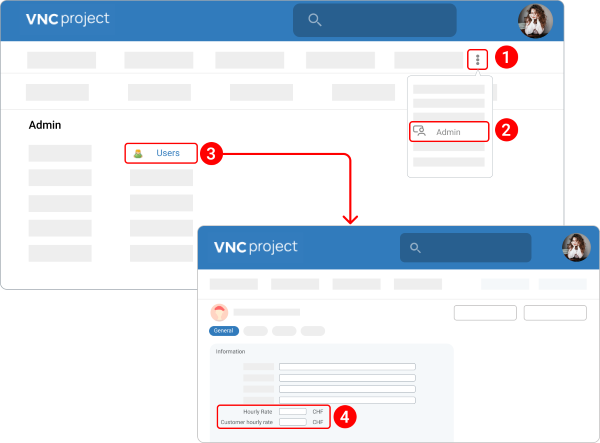For example, the user Mia Chesterfield is set to a basic hourly rate of 30 CHF and a customer hourly rate of 50 CHF.

We have the option to set the initial hourly rate via the first history item containing the hourly rate change. This rate will be used for the time logs created before we have set the hourly rate for the user. This option is also available for the “Customer hourly rate”.

In the same example, we see that the customer's hourly rate was changed from 30 CHF to 50 CHF. But we can find the old initial hourly rate if we click the pencil icon.

If there is no history item for any rate, then the current profile rate is used for time logged.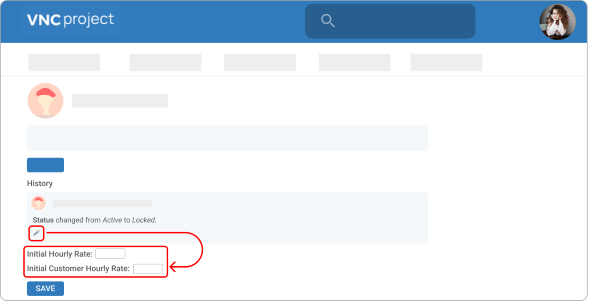### Define EVM Values in a project¶

1. Open a required project.
2. Under the Description section, click Settings.
3. Click the down arrownext to Advanced Settings.
4. Under the EVM data section, you can customize the following fields:

• Contract Value
• Desired Profit
• Minimum Good Profit
• Minimum Acceptable Profit
• Rate multiplier for customers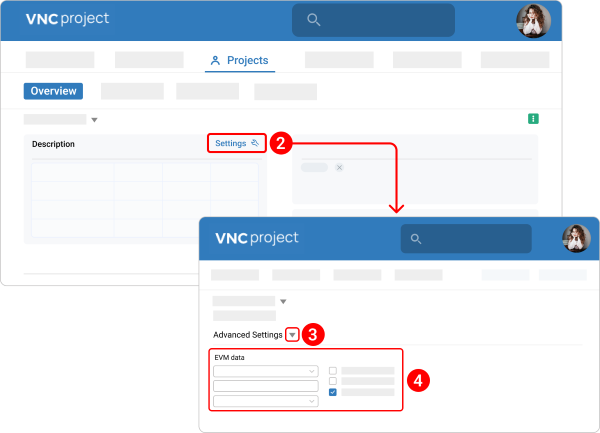The contract value is the contractually agreed price, which was signed with the customer, or the planned value for the internal project, which can be added by the project manager. The contract values in VNCproject are set to CHF in our example.

Three percentage profit values are defined at the project level: “Desired Profit”, “Minimum Good Profit” and “Minimum Acceptable Profit”.

Rate multiplier for customers: The internal calculations are based on the basic hourly rate of the employees, but in the customer project we use an external rate (customer hourly rate) to enhance the profit. So the “rate multiplier” is used to calculate the external rate based on the internal rate, following this formula:

External rate = Rate multiplier for customers x Internal rate

In the example below, the contract value of the vnc-demo-project is 3.000 CHF. The percentage of “Desired Profit”, “Minimum Good Profit” and “Minimum Acceptable Profit” are defined with a ratio of 40/30/20. That means: - Desired Profit = 40% of 3.000 CHF = 1.200 CHF - Minimum Good Profit = 30% of 3.000 CHF = 900 CHF - Minimum Acceptable Profit = 20% of 3.000 CHF = 600 CHF

The rate multiplier for customers = 3. If one of the employees' rates is 10 CHF per hour, then the external EVM calculations for this employee will be 30 CHF, and all EVM customer calculations will be based on this external rate.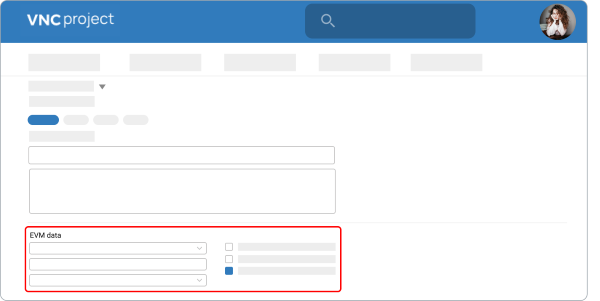### Define multiple contract values for a project¶

In some projects, there are different contract values per period.

1. Open a required project.
2. Under the Description section, click Settings.
3. On the top you can navigate between the different project settings. Select the Contract Values chip.
4. Click the NEW CONTRACT VALUE button.
5. Give a contract value a name.
7. Define the start date and end date.
8. Click CREATE.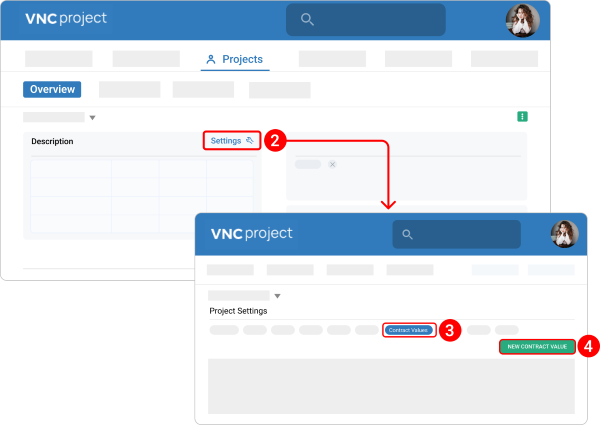For each contract value, tickets created between these dates will be considered.

On the project overview, the sum of all contract values will be considered as project contract value. We can see the EVM data for a particular contract value period on the EVM Report tab.

The percentage profit values are defined at the project level and will be used for each contract value per period.

Validation:

• Contract value should be greater than 0
• Start date must be greater than the previous contract's End date.
• End date must be greater than the Start date.
• Start date and End date range must be exclusive of other contract values defined in the project

Any updating/creation/deletion of contract values will update the project contract value. There are two more columns in the contract's setting: One is “Description”, in which the manager can add more details about each contract. The “Date” column will show the created date and time of each contract and its last update.

For example:

In this project, we have four different contract periods from the 1st of April 2023 until the 21st of June 2023 with a total contract value of 2.800 CHF:

From 04/01/2023 to 04/15/2023 & from 04/16/2023 to 04/30/2023: 500 CHF each time
From 05/01/2023 to 05/31/2021: 1.000 CHF
From 01/06/2021 to 21/06/2021: 800 CHF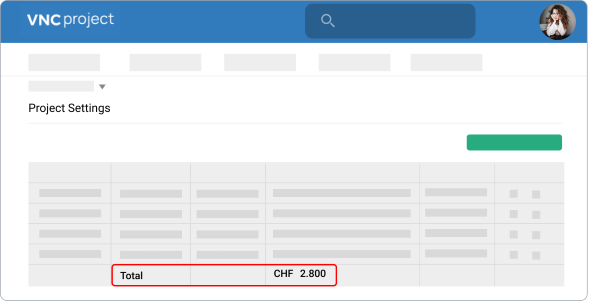### Define purchase orders for a project¶

Besides the multiple contracts, we can create also purchase orders for each version.

1. Open a required project.
2. Under the Description section, click Settings.
3. On the top you can navigate between the different project settings. Select the Purchase Orders chip.
4. Click the NEW PURCHASE ORDER button.
5. Add all the order's details such as Issue's number, Version's name, Order's number, Amount, Date, and Description.
6. In the mandatory field “PDF file”, we can add any files, which relate to the order in PDF format.
7. Click CREATE.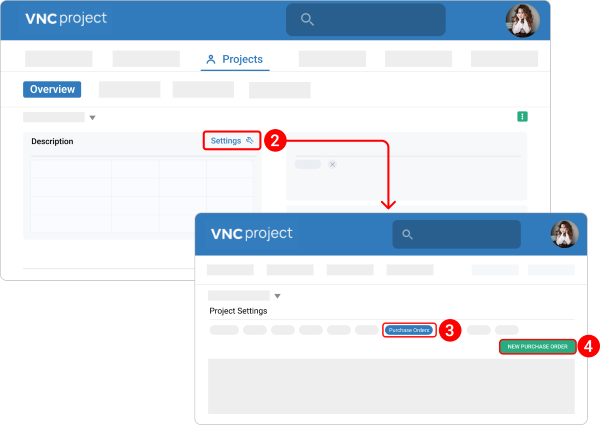After setting, all details of the PO will be shown in the Purchase Orders tab. The sum of all purchase order values should be equal to the total values of the project's contract. If this is not the case, a warning stating Sum of all purchase orders values is not equal to the total values of the project's contract.

### Define Cost Items¶

You can define a new type of “Cost Item”. It will be used to log the “Other costs” at the ticket level. For example, you can define a new type of cost for “Travel Expenses”.

1. In the navigation menu, click the kebab menu icon.
3. Click Cost Items.
4. Click the NEW COST ITEM button.
5. Give a cost item a German and English name.
7. Check the box next to Active.
8. Click CREATE.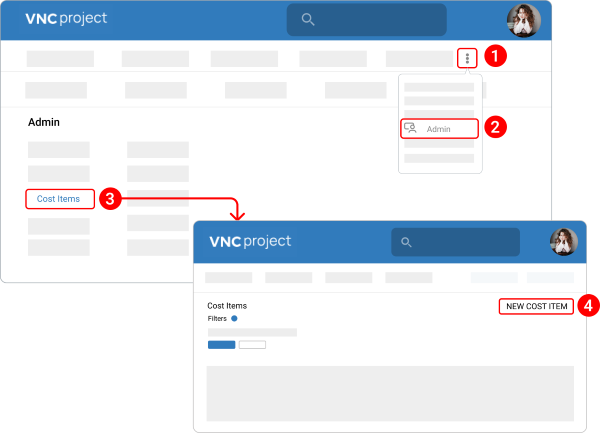After the settings of “Cost items”, we can add the values of “Estimated cost items” or “Spent cost items” to the ticket’s page. In the example below, 5 CHF for “Bus ticket” is added to “Spent cost items”: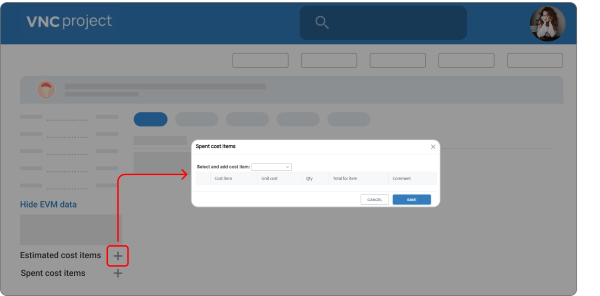Then these “traveling expenses” will be displayed in the "Other Costs" on the ticket’s page. The values of “Estimated cost items” after defining will be displayed in “Budget of other Costs”:

## Calculation EVM¶

### EVM at a ticket level¶

#### EVM values for main ticket and sub tickets¶

1. Estimated resource cost = (Time logged by user1 × user1 rate on time log date) + (Time logged by user2 × user 2 rates on time log date) + ... + (Time logged by user N × user N rate on time log date) + (Remaining time × current assignee’s current rate if there is any remaining time)

2. Actual Resource log time Cost = (Time logged by user1 × user 1 rate on time log date) + (Time logged by user2 × user2 rate on time log date) + ... + (Time logged by user N × user N rate on time log date)

3. Calculation of "Planned Value" (PV) in ticket level: Planned value is the budget planned until today and it bases on setting the start date, due date, duration (between the start date and due date), and the estimated cost per day or the BC.

• 1. Case: today's date is less than the due date

PV = Number of day since reported × Estimated cost per day
Estimated cost per day = BC / ticket's duration

• 2. Case: today's date is greater than the due date, then PV = BC**

The progress (% Done) will be calculated on multiple levels of the project with the bottom-up principle: from every single task (the lowest level) to the tickets level, version level, and finally to the project's level.

Other EVM formulas to calculate the BC, AC, EV, CV, CPI, EAC, SV, and “Expected profit” in ticket level will be shown in the picture below.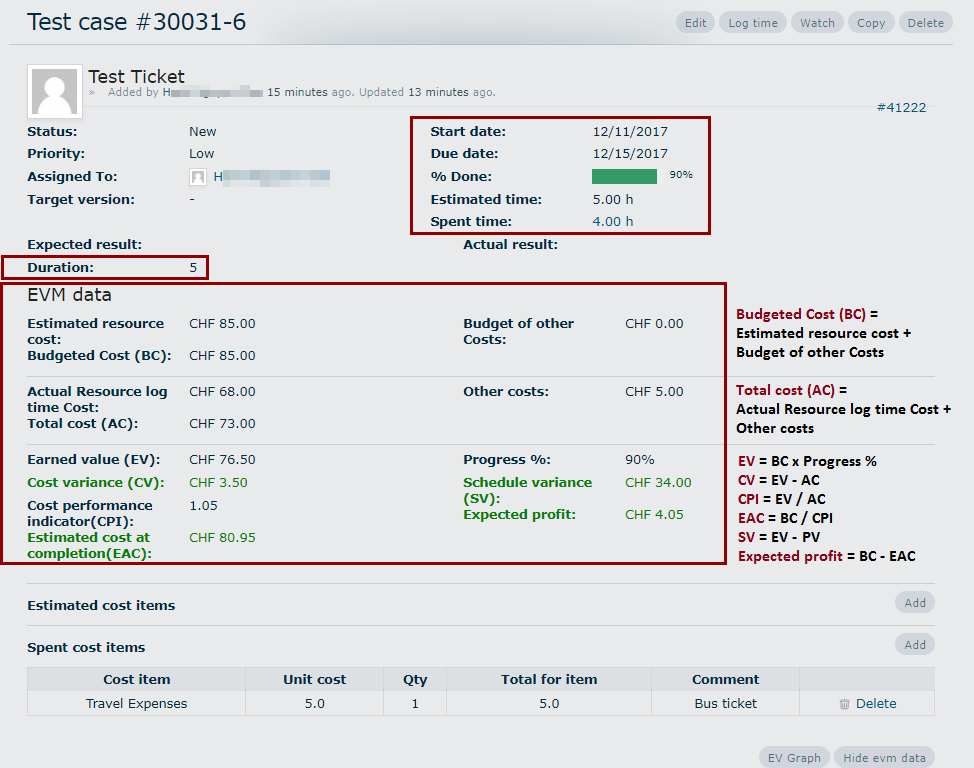In this example, the PV is calculated with the formula in the first case because the number of days since reported is 2.5 days (today's date is less than the due date)

Estimated cost per day = BC / ticket's duration = 85 / 5 = 17 (CHF per day)

PV = Number of days since reported × Estimated cost per day = 2.5 x 17 = 42.5 CHF.

• EVM Graph

We can click the button “EV Graph” on the right bottom to see the EV Graph of the ticket.

If the red line of EV is above the blue line of PV or above the orange line of AC, the ticket’s cost is under the budget and ahead of schedule. If the red line of EV is under the blue line or under the orange line, the ticket’s cost is over the budget and behind schedule. In this case, the red line of EV is identical to the blue line of PV or the orange line of AC, the ticket’s cost is on the budget and on schedule.

The green line in this graph will show the schedule variance (SV). If this line is above/under the x-axis (0-line), the ticket’s cost is ahead/behind schedule.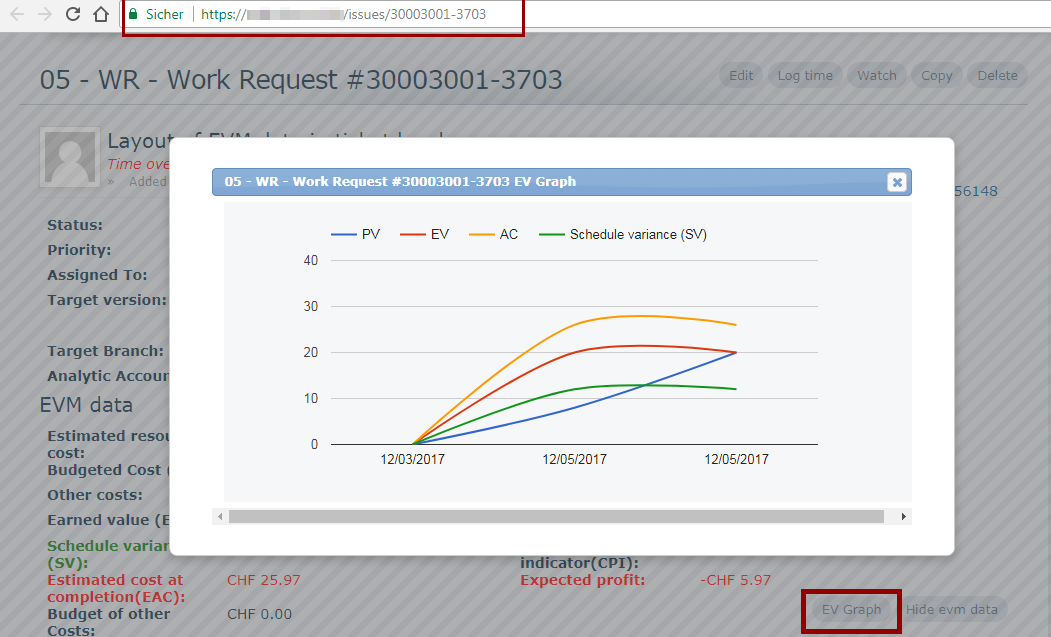### Quick EVM performance¶

There is a quick performance on the project overview page to show the important values of EVM. The people who have access to EVM can see how the project is doing on the main page of the project.

Before checking the EVM values on the main page, we need to calculate EVM data to see the actual result. Without “Calculate EVM data” the system computes EVM data automatically at 5 AM every day.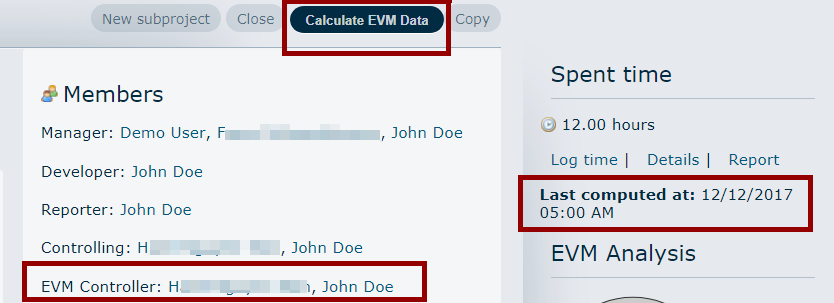There are 3 different quick performances on the main page: an EVM Bar Chart on the left side of the page, an EVM Graph in the middle, and a quick EVM sidebar on the right side with a “profit scale.EVM sidebar:The total EVM calculation for the project’s level on a certain date will be shown in the picture below.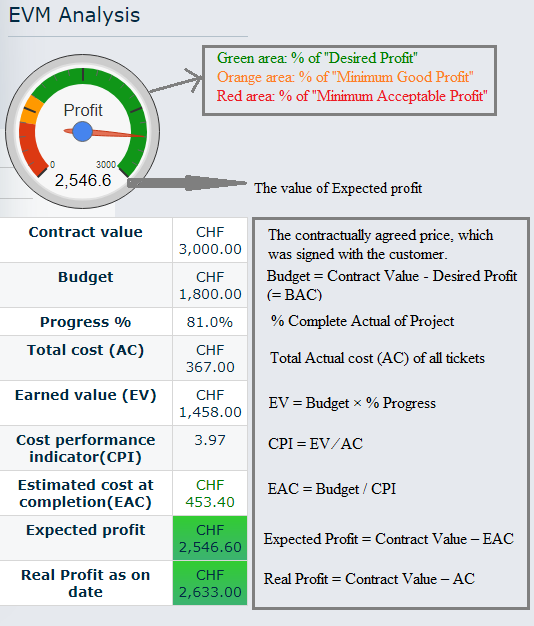EVM Graph: This “EVM Graph” on the project’s main page shows the same 3 basic factors as the “EVM Graph” on the issue’s page. The difference is, the budget (BC), EV, and AC in this graph are the values of the project’s level from the beginning until the computed date. A positive result will be realized if the red line of EV is above the blue line of BC or the orange line of AC (under the budget and ahead of the schedule). If not then the project has a negative result (over the budget and behind the schedule).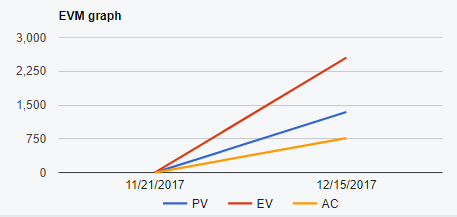EVM Bar Chart:The total BC, EV, and AC of the project as of date will be shown in the “EVM Bar Chart”. If the “% Progress” of the project reaches 100 %, the total EV will be equal to the BC.### EVM Report¶

The full EVM Report will be shown in the “EVM Report” tab. There are 4 tabs inside this tab: Overview, Report, Versions details, and Tickets details.

1. Overview:
In this tab, we can see the EVM values at the project’s level and its “Bar Chart”.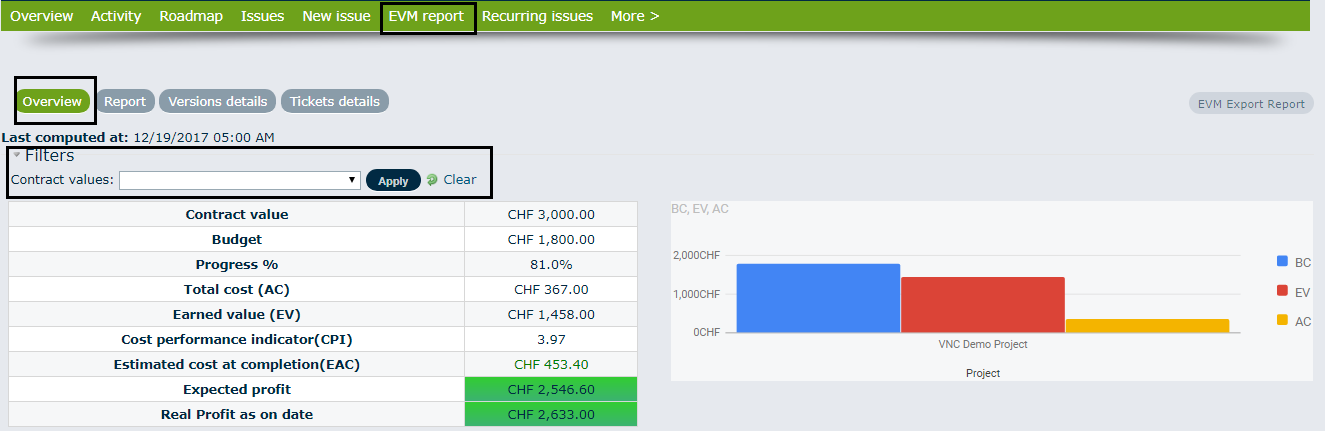If the project has multiple contract values, we can select the contract value by using the “Filters” function: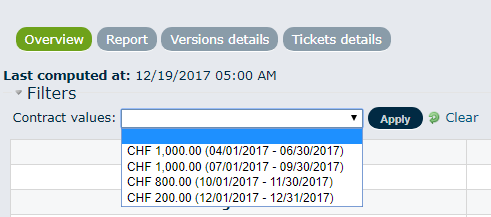For example, we choose the contract for the period from 01.04.2017 to 30.06.2017, all the EVM values and the bar chart will be filtered and changed within the respective contract’s period.

Please note: The filtered contract’s period has only 2 tabs to display, the “Overview” and the “Tickets details”.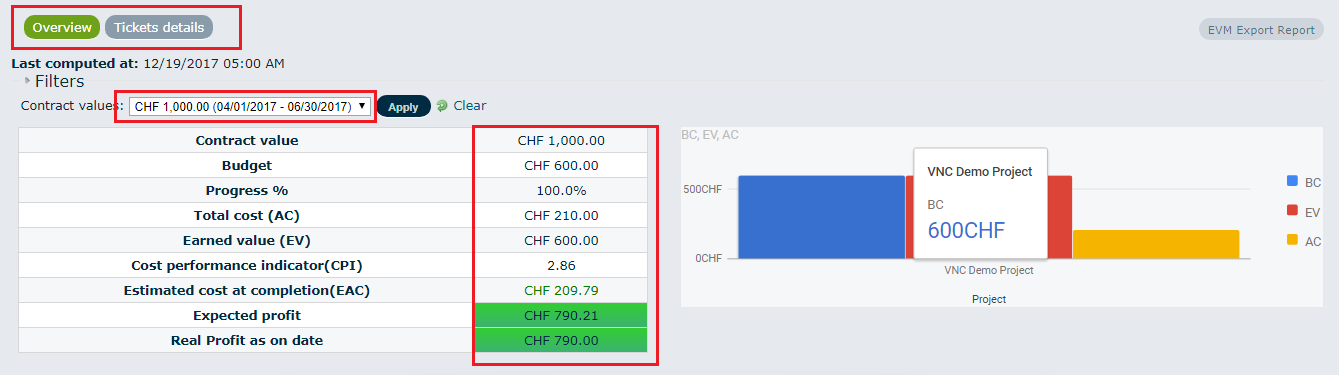2. Report:

In this tab, we can see and compare the EVM values monthly. We can see again the “EVM Graph” with 3 factors PV, AC, and EV. If we click in some places in the line, the value of the month will be displayed.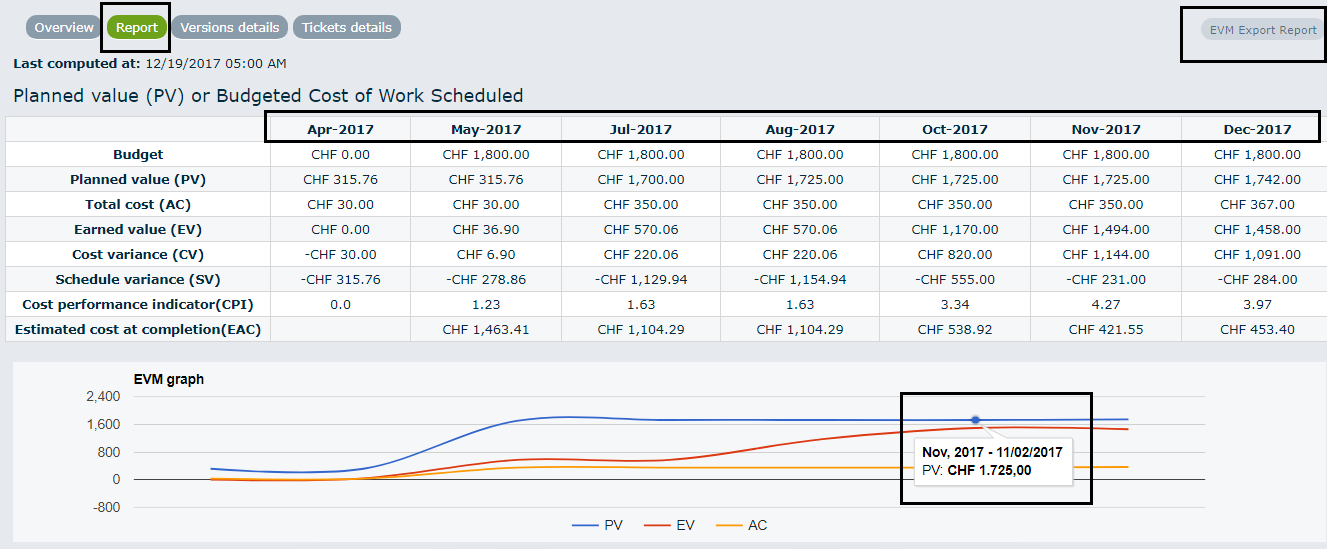3. Versions details:

In this tab, all the version details and non-version details with the total values of each EVM factor will be displayed. In the example below, there are 2 versions without total cost values. The CPI is 0 and the EAC will not be identified.

We can also compare the “contract value” of each version (according to the purchase order) and the actual budget of each version (based on the project's budget). The value of non-version is zero.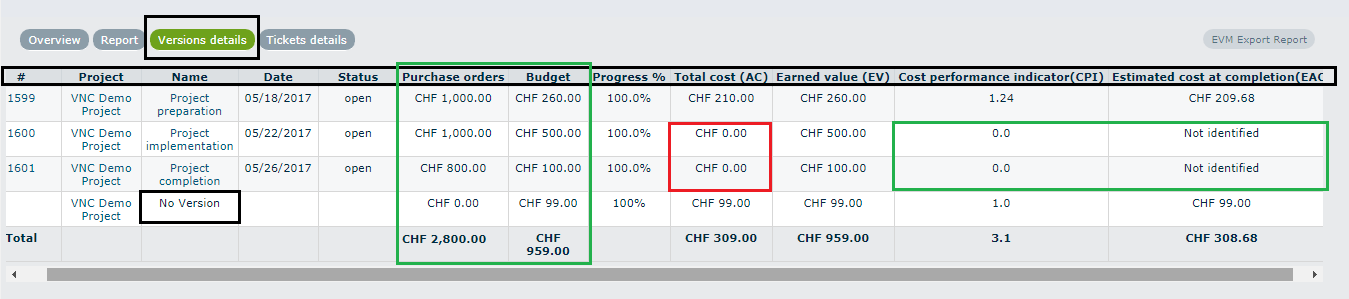4. Tickets details:

The last tab in the EVM Report is the “Tickets Details” tab. In this tab, all tickets will be displayed in detail. To see the details easier, we can use the “Options” function to select any respective column. The last row in the table is the total values of all tickets.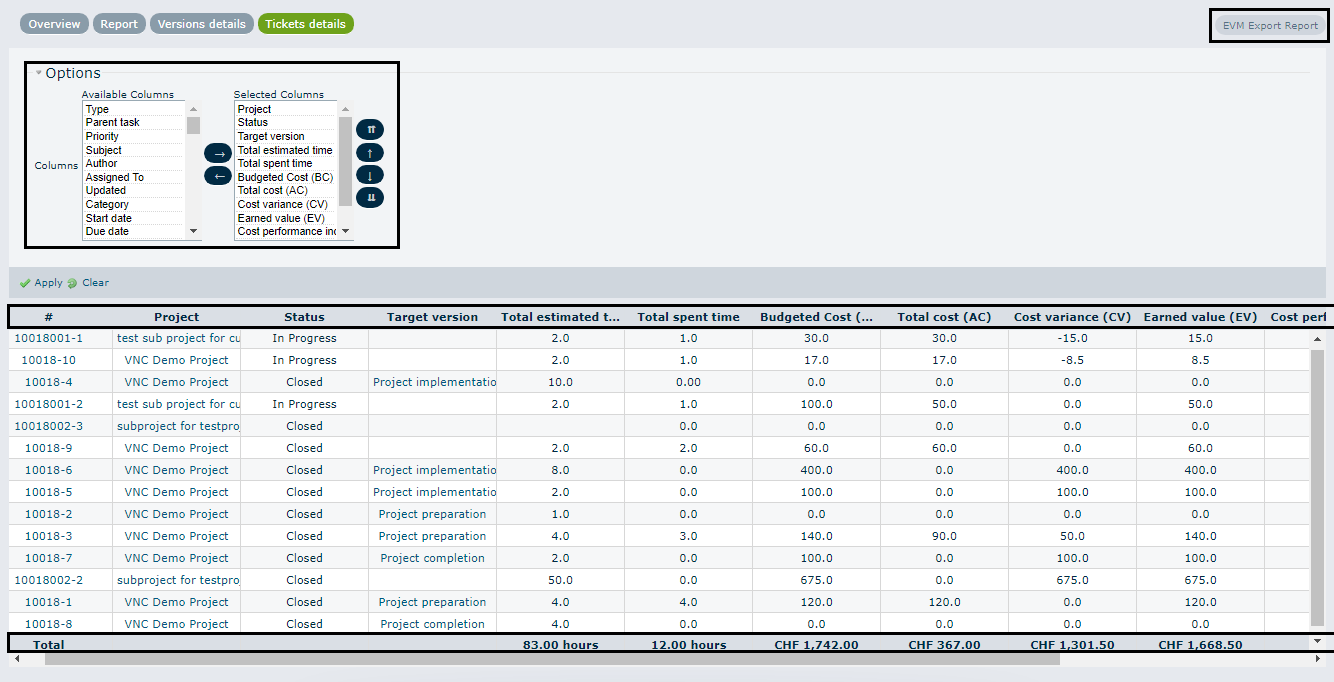At any point in time, we can download a full detailed report of the EVM data, if we click the “EVM export report” on the top-right of the screen. The EVM sheet will include all the values of EVM related to the project.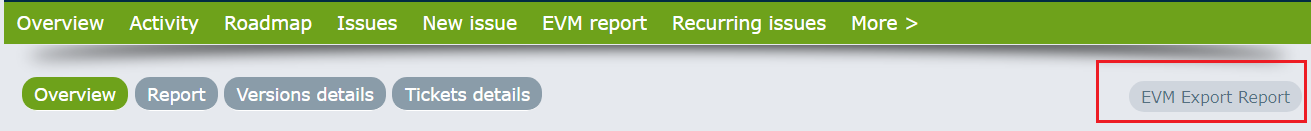### Overview of the EVM values for all projects¶

The following values of EVM should appear also in the overview of all projects: Budget (BAC), Progress %, Total cost (AC), Earned value (EV), Cost variance (CV), Schedule Variance (SV), Estimated cost at completion (EAC) and Expected Profit.

With the “Filters” and “Options” functions we can select any kind of projects or any columns as required.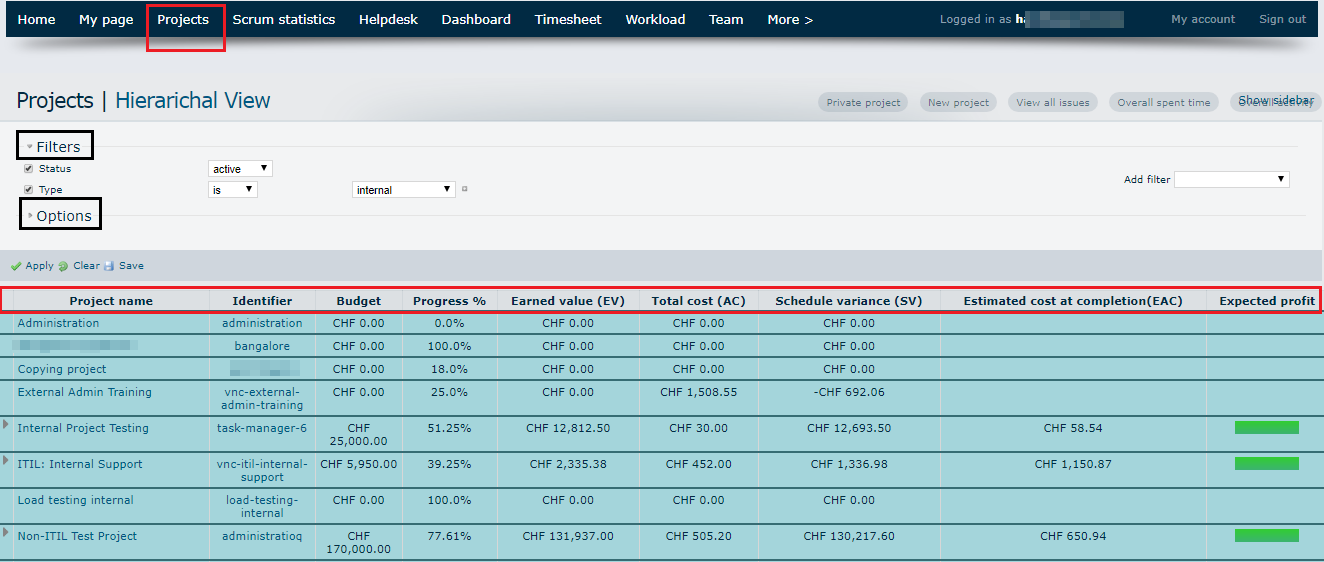### The EVM at the Dashboard¶

We can choose the EVM with the specific value on the dashboard. All the projects, which are based on these filtered values will be shown. In the example below, all projects with “Expected profit” greater than 0 and their “% Progress” greater than 50% are filtered.